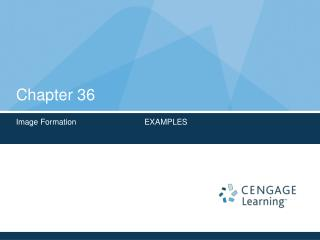DownloadDownload PresentationChapter 36

Chapter 36

Télécharger la présentationChapter 36

- - - - - - - - - - - - - - - - - - - - - - - - - - - E N D - - - - - - - - - - - - - - - - - - - - - - - - - - -
Presentation Transcript

1. Chapter 36 Image Formation EXAMPLES

2. Chapter 36 Image Formation: Examples

3. Example 36.1 Multiple Images Formed by Two Mirrors • Two flat mirrors are perpendicular to each other. An object is placed at point O. How many images do you see? • Image I1 at mirror 1 • Image I2 at mirror 2 • Image I3 : I1 reflected in mirror 1, or • I2 reflected in mirror 2. • Formula to find the number of images for any angle  between the mirrors

4. Example 36.2 The Tilting Rearview Mirror • With the daytime setting, the bright beam of reflected light is directed into the driver’s eyes • With the nighttime setting, the dim beam of reflected light is directed into the driver’s eyes, while the bright beam goes elsewhere

5. Example 36.3 The Image Formed by a Concave Mirror • A spherical mirror has a focal length of +10cm (A). Locate and describe the image of an object distance of 25.0 cm (p > R) Image is real, smaller than the object, inverted and located on the front side of the mirror

6. Example 36.3 The Image Formed by a Concave Mirror, 2 (B). Locate and describe the image of an object distance of 10 cm. (Object is located at the focal length p = f) Image is extra-larger than the objet and formed at an infinite distance from the mirror.

7. Example 36.3 The Image Formed by a Concave Mirror, final (C). Locate and describe the image of an object distance of 5.0 cm (p < f) Image is virtual, upright, larger than the objet and formed on the back side of the mirror.

8. Example 36.4 Gaze Into the Crystal Ball • A set of coins is embedded in a spherical plastic paper weight of radius 3.0 cm, with n = 1.50. One coin is located 2.0 cm from the edge of the sphere. Find the position of the image of the coin. • Here: n1 > n2 so a virtual image is formed inside the paperweight. • R is negative

9. Example 36.5 Catch the Fish • A small fish is swimming at a depth d below the surface of a pond. (A). What is the apparent depth of the fish as viewed from directly overhead? Here: n1 > n2. Because the refracting surface is flat, R is infinite. Then using Eqn. 36.8 (with p = d) we can find the location of the image (q). Then : Because q is negative, the image is virtual as indicated by the dashed green line

10. Example 36.5 Catch the Fish, final (B). If your face is a distance dabove the water surface, at what apparent distance above the surface does the fish see your face? Similar to part (A). Because the refracting surface is flat R is infinite. Then using Eqn. 36.8 (with p = d) we can find the location of the image (q). Then: , so: The negative sign for q indicates that the image is in the medium from which the light originated (the air)

11. Example 36.6 Images Formed by a Converging Lens • A converging lens has a focal length of +10cm (A). An object is placed 30.0cm from the lens. Construct a ray diagram, to find the image distance, and describe the image. Image is real, smaller than the object, inverted and located on the back side of the lens

12. Example 36.6 Images Formed by a Converging Lens, 2 (B). Locate and describe the image of an object placed 10.0cm from the lens. (Object is located at the focal length p = f) Image is extra-larger than the objet and formed at an infinite distance from the lens.

13. Example 36.6 Images Formed by a Converging Lens, final (C). An object is placed 5.0cm from the lens. Construct a ray diagram, to find the image distance, and describe the image. Image is virtual, upright, larger than the objet and formed on the front side of the lens.

14. Example 36.7 Where is the Final Image?

15. Example 36.7 Where is the Final Image?, final • The location of the image formed by lens 1 • The image of lens 1 becomes object for lens 2, with p2 = 20cm – 15cm = 5cm  • Then, the total magnification will be:

16. Material for the Midterm • Material from the book to Study!!! • Objective Questions: 9-12 • Conceptual Questions: 1-6 • Problems:3-9-14-28-35-41-42-43-44